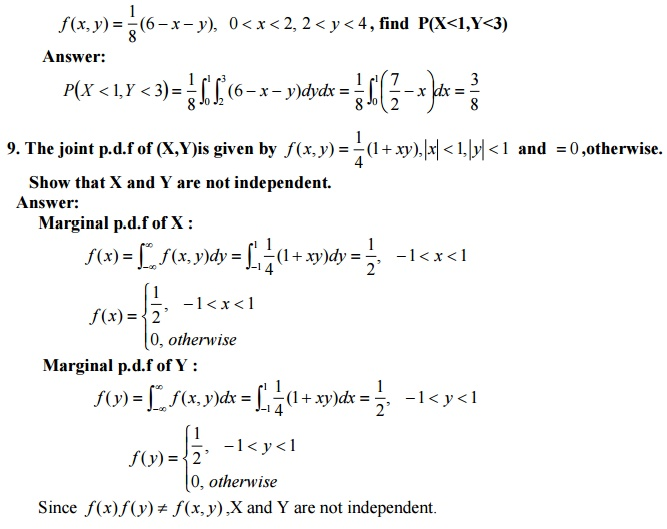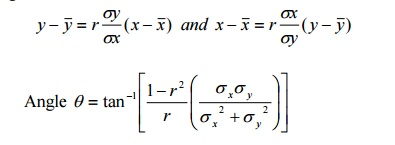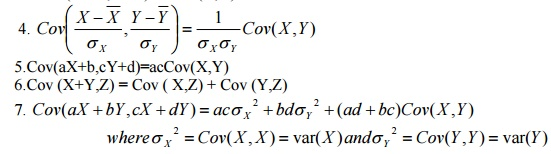Home | | Probability and Statistics | Important Short Objective Questions and Answers: Two Dimensional Random Variables

# Important Short Objective Questions and Answers: Two Dimensional Random Variables

Mathematics (maths) - Two Dimensional Random Variables - Important Short Objective Questions and Answers: Two Dimensional Random Variables

Two Dimensional Random Variables

1.Define Two-dimensional Random variables.

Let S be the sample space associated with a random experiment E. Let X=X(S) and Y=Y(S) be two functions each assigning a real number to each sS. Then (X,Y) is called a two dimensional random variable.

2.The following table gives the joint probability distribution of X and Y. Find the mariginal density functions of X and Y.

Y /X  1        2        3

1        0.1     0.1     0.2

2        0.2     0.3     0.13. If f (x, y)= kxye(x2 + y2 ) ,  x ≥ , y0 ≥ 0    is the joint pdf, find k .5. The joint p.m.f of (X,Y) is given by P(x, y) = k(2x + 3y), x = ,1,;02y = ,13,.2.Find the marginal probability distribution of X.6. If X and Y are independent RVs with variances 8and 5.find the variance of 3X+4Y. Answer:

Given Var(X)=8 and Var(Y)=5

To find: var(3X-4Y)

We know that Var(aX - bY)=a2Var(X)+b2Var(Y) var(3X- 4Y)=32Var(X)+42Var(Y) =(9)(8)+(16)(5)=152

7.Find the value of k if f (x, y) = k(1 x)(1 y) for0 < x, y < 1 is to be joint density function.8. If X and Y are random variables having the joint p.d.f11. The joint p.d.f of two random variables X and Y is given by13. If the joint pdf of (X,Y) is  f (x, y) = 6e−2x−3y , x ≥ , y0 ≥ , find0 the conditional density  of Y given X.

Given f (x, y) = 6e−2x−3y , x ≥ , y0 ≥  , 0

The Marginal p.d.f of X:14.Find the probability distribution of (X+Y) given the bivariate distribution of (X,Y).15. The joint p.d.f of (X,Y) is given by f (x, y) = 6e(x+ y) , 0 x, y ≤ ∞.Are X and Y independent?f (x) f (y) = f (x, y)

X and Y are independent.

16. The joint p.d.f of a bivariate R.V (X,Y) is given by17. Define Co – Variance:

If X and Y are two two r.v.s then co – variance between them is defined as Cov (X, Y) = E {X – E (X)} {Y – E (Y)}

(ie) Cov (X, Y) = E (XY) – E(X) E (Y)

18.State the properties of Co – variance;

1. If X and Y are two independent variables, then Cov (X,Y) = 0. But the

Converse need not be true

2.Cov (aX, bY) = ab Cov (X,Y)

3.Cov (X + a,Y +b) = Cov (X,Y)5.Cov(aX+b,cY+d)=acCov(X,Y)

6.Cov (X+Y,Z) = Cov ( X,Z) + Cov (Y,Z)

7. Cov(aX + bY,cX + dY) = acσ X 2 + bdσY 2 + (ad + bc)Cov(X ,Y) whereσ X 2 = Cov(X , X ) = var(X )andσY 2 = Cov(Y,Y) = var(Y)

Take U= aX+b and V= cY+d

Then E(U)=aE(X)+b and E(V)= cE(Y)+d U-E(U)= a[X-E(X)] and V-E(V)=c[Y-E(Y)]

Cov(aX+b,cY+d)= Cov(U,V)=E[{U-E(U)}{V-E(V)}] = E[a{X-E(X)} c{Y-E(Y)}] =ac E[{X-E(X)}{Y-E(Y)}]=acCov(X,Y)

20.If X&Y are independent R.V’s ,what are the values of Var(X1+X2)and Var(X1-X2) Answer:

Var(X1 ± X2 =) Var(X1 +) Var(X2 )(Since X andY are independent RV then Var(aX ± bX) = a2Var(X) + b2Var(X) )

21.If Y1& Y2 are independent R.V’s ,then covariance (Y1,Y2)=0.Is the converse of the above statement true?Justify your answer.

The converse is not true . Consider

X - N(0,1)and Y = X2  sin ceX N( 1,),0

E(X ) = ; 0E(X 3 ) = E(XY) = 0sin ce all odd moments vanish.

cov(XY) = E(XY) E(X )E(Y) = E(X 3 ) E(X )E(Y) = 0

cov(XY) = 0 but X &Y areindependent

22.Show that cov2 (X ,Y) var(X ) var(Y)

cov(X ,Y) = E(XY) E(X )E(Y) We know that [E(XY)]2 E(X 2 )E(Y 2 )

cov2 (X ,Y) = [E(XY)]2 + [E(X )]2 [E(Y)]2 2E(XY)E(X )E(Y)

E(X )2 E(Y)2 + [E(X )]2 [E(Y)]2 2E(XY)E(X )E(Y)

E(X )2 E(Y)2 + [E(X )]2 [E(Y)]2 E(X 2 )E(Y)2 E(Y 2 )E(X )2

= {E(X 2 ) − [E(X )]2 }{E(Y 2 ) − [E(Y)]2 }≤ var(X ) var(Y)

23.If X and Y are independent random variable find covariance between X+Y and X-Y. Answer:

cov[X + Y, X Y ]= E[(X + Y)(X Y)]− [E(X + Y)E(X Y)]

= E[X 2 ] E[Y 2 ] [E(X )]2 + [E(Y)]2

= var(X ) var(Y)

24.X and Y are independent random variables with variances 2 and 3.Find the variance 3X+4Y.

Given var(X) = 2, var(Y) = 3

We know that  var(aX+Y) = a2var(X) + var(Y)

And   var(aX+bY) = a2var(X) + b2var(Y)

var(3X+4Y) = 32var(X) + 42var(Y) = 9(2) + 16(3) = 66

25. Define correlation

The correlation between two RVs X and Y is defined as26. Define uncorrelated

Two RVs are uncorrelated with each other, if the correlation between X and Y is equal to the product of their means. i.e.,E[XY]=E[X].E[Y]33. State the equations of the two regression lines. what is the angle between them? Answer:

Regression lines:34. The regression lines between two random variables X and Y is given by 3X + Y = 10 and3X + 4Y = 12 .Find the correlation between X and Y.35.Distinguish between correlation and regression.

By correlation we mean the casual relationship between two or more variables. By regression we mean the average relationship between two or more variables.

36. State the Central Limit Theorem.standard normal distribution as n → ∞ provided the m.g.f of xi exist.

37.The lifetime of a certain kind of electric bulb may be considered as a RV with mean 1200 hours and S.D 250 hours. Find the probability that the average life time of exceeds 1250 hours using central limit theorem.

Solution:

Let X denote the life time of the 60 bulbs.

Then µ = E(X)= 1200 hrs. and Var(X)=(S.D)2= σ2=(250)2hrs.38.Joint probability distribution of (X, Y)

Let (X,Y) be a two dimensional discrete random variable. Let P(X=xi,Y=yj)=pij. pij is called the probability function of (X,Y) or joint probability distribution. If the following conditions are satisfied

1.pij 0  for all i and jThe set of triples (xi,yj, pij) i=1,2,3……. And j=1,2,3……is called the Joint probability distribution of (X,Y)

39.Joint probability density function

If (X,Y) is a two-dimensional continuous RV such thatThen f(x,y) is called the joint pdf of (X,Y) provided the following conditions satisfied.

f (x, y) 0 for all (x, y)(−∞,)40.Joint cumulative distribution function (joint cdf)

If (X,Y) is a two dimensional RV then F(x, y) = P(X x,Y y) is called joint cdf of (X,Y) In the discrete case,41.Marginal probability distribution(Discrete case )

Let (X,Y) be a two dimensional discrete RV and pij=P(X=xi,Y=yj) thenis called the Marginal probability function.

The collection of pairs {xi,pi*} is called the Marginal probability distribution of X.then the collection of pairs {xi,p*j} is called the Marginal probability distribution of Y.

42.Marginal density function (Continuous case )

Let f(x,y) be the joint pdf of a continuous two dimensional RV(X,Y).The marginal density43.Conditional probability function

If pij=P(X=xi,Y=yj) is the Joint probability function of a two dimensional discrete RV(X,Y)

then the conditional probability function X given Y=yj is defined byThe conditional probability function Y given X=xi is defined by

44.Conditional density  function

Let f(x,y) be the joint pdf of a continuous two dimensional RV(X,Y).Then the Conditional  density function of X given Y=y is defined by  f (X Y) = fXY (x, y) /fY (y) ,where f(y)=marginal45.Define statistical properties

Two jointly distributed RVs X and Y are statistical independent of each other if and only if the joint probability density function equals the product of the two marginal probability density function

i.e., f(x,y)=f(x).f(y)

46.The joint p.d.f of (X,Y) is given by f (x, y) = e(x+ y)  0 x, y ≤ ∞ .Are X and Y are

independent?X and Y are independent since f(x,y)=f(x).f(y)

47.Define Co – Variance :

If X and Y are two two r.v.s then co – variance between them is defined as Cov (X, Y) = E {X – E (X)} {Y – E (Y)}

(ie) Cov (X, Y) = E (XY) – E(X) E (Y)

48.             State the properties of Co – variance;

1. If X and Y are two independent variables, then Cov (X,Y) = 0. But the

Converse need not be true

2.Cov (aX, bY) = ab Cov (X,Y)

3.Cov (X + a,Y +b) = Cov (X,Y)Take U= aX+b and V= cY+d

Then E(U)=aE(X)+b and E(V)= cE(Y)+d U-E(U)= a[X-E(X)] and V-E(V)=c[Y-E(Y)]

Cov(aX+b,cY+d)= Cov(U,V)=E[{U-E(U)}{V-E(V)}] = E[a{X-E(X)} c{Y-E(Y)}] =ac E[{X-E(X)}{Y-E(Y)}]=acCov(X,Y)

Study Material, Lecturing Notes, Assignment, Reference, Wiki description explanation, brief detail
Mathematics (maths) : Two Dimensional Random Variables : Important Short Objective Questions and Answers: Two Dimensional Random Variables |